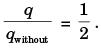Courses

## 23 Questions MCQ Test Engineering Mechanics | Past Year Questions: Radiation

Description
This mock test of Past Year Questions: Radiation for Mechanical Engineering helps you for every Mechanical Engineering entrance exam. This contains 23 Multiple Choice Questions for Mechanical Engineering Past Year Questions: Radiation (mcq) to study with solutions a complete question bank. The solved questions answers in this Past Year Questions: Radiation quiz give you a good mix of easy questions and tough questions. Mechanical Engineering students definitely take this Past Year Questions: Radiation exercise for a better result in the exam. You can find other Past Year Questions: Radiation extra questions, long questions & short questions for Mechanical Engineering on EduRev as well by searching above.
QUESTION: 1

### For a glass plate transmissivity and reflectivity are specified as 0.86 and 0.08 respectively, the absorptivity of the glass plate is 

Solution: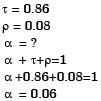QUESTION: 2

Solution:

QUESTION: 3

### What is the value of the view factor for two inclined flat plates having common edge of equal length and wlth an angle of 20 degrees? 

Solution: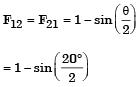= 0.826

QUESTION: 4

The following figure was generated from experimental data relating spectral black body emissive power to wave length at the three temperatures T1, T2 and T3( T1 > T2 >T3).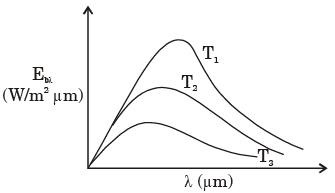The conclusion in that the measurements are



Solution:

Wien's displacement law is not satisfied, i.e., λmax T = C
which tells lower λmax for higher temperature.

QUESTION: 5

For an opaque surface, the absorptivity (α), transmissivity (τ) and reflectivity (ρ) are related by the equation



Solution:

α + τ + ρ = 1
for opaque surface, τ = 0
∴ α + ρ = 1

QUESTION: 6

The radiative heat transfer rate per unit area (W/m2) between two plane parallel gray surfaces (emissivity = 0.9) maintained at 400 K and 300 K is (σ = Stefan Boltzmann constant = 5.67 × 10-8 W/m2K4)



Solution:

For plane surfaces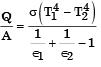Here ∈1 = ∈2 = 0.9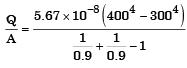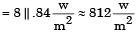QUESTION: 7

A plate having 10 cm2 area each side is hanging in the middle of a room of 100 m2 total surface area. The plate temperature and emissivity are respectively 800 K and 0.6. The temperature and emissivity valute for the surfaces of the room are 300 K and 0.3 respectively. Boltzmann's constant σ = 5.67 × 10-8 W/m2K4. The total heat loss from the two surfaces of the plate is



Solution:

Heal loss from one surface of the plate,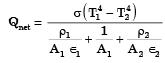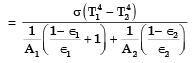Putting
A1 = 10 × 10–4 m2, T1 = 800
1 = 0.6 T2 = 300
A2 = 100 m2
2 = 0.3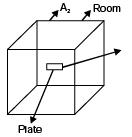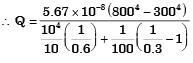= 13.66 W
∴ Heat loss from both the surfaces
= 2 Qnet
= 2 × 13.66 = 27.32 W

QUESTION: 8

A100 W electric bulb was switched on in a 2.5 m × 3 m × 3 m size thermally insulated room having a temperature of 20°C. The room temperature at the end of 24 hours will be



Solution:

Heat generated by bulb = 100 × 24 × 60 × 60 Joule.
∴ Heat dissipated = r × V × Cv × (T - 20)
⇒ 100 × 24 × 60 × 60 = 1.24 × 9 × 2.5 × 3 × Cv (T – 20)
⇒ T = 450º

QUESTION: 9

Radiative heat transfer is intercepted between the inner surfaces of two very large isothermal parallel metal plates. While the upper plate (designated as plate 1) is a black surface and is the warmer one being maintained at 727°C, the lower plate (plate 2) is a diffuse and gray surface with an emissivity of 0.7 and is kept at 227°C. Assume that the surfaces are sufficiently large to form a two-surface enclosure and steady-state conditions to exist. Stefan-Boltzmann constant is given as 5.67 × 10–8 W/m2K4.

The irradiation (in kW/m2) for the plate (plate 1) is



Solution:

T1 = 1000 K
T2 = 500 K
∈ = 0.7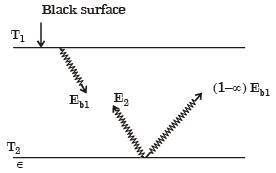Energy indicent upon plate 1 due to irradiation = Radiosity of plate 2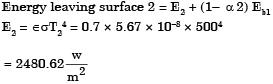σ (1- α) Eb1 = Fraction of energy reflected from surface 2
= (1 – 0.7) × 5.67 × 10–8 ×10004
= 17010(W/m2)
∴ Total energy incident upon plate 1 =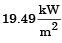QUESTION: 10

Radiative heat transfer is intercepted between the inner surfaces of two very large isothermal parallel metal plates. While the upper plate (designated as plate 1) is a black surface and is the warmer one being maintained at 727°C, the lower plate (plate 2) is a diffuse and gray surface with an emissivity of 0.7 and is kept at 227°C. Assume that the surfaces are sufficiently large to form a two-surface enclosure and steady-state conditions to exist. Stefan-Boltzmann constant is given as 5.67 × 10–8 W/m2K4.

If plate 1 is also a diffuse and gray surface with an emissivity value of 0.8, the net radiatibn heat exchange (in kW/m2) between plate 1 and plate 2 is



Solution: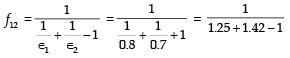= 0.59
∴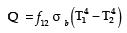= 0.59 x 5.67 x (10000 - 625)
= 31362.18 W/m2
= 31.36 kW/m2
Alternately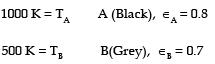Net heat exchange between A and B,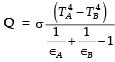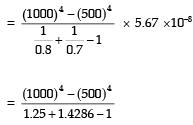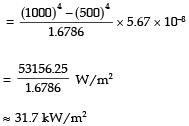QUESTION: 11

Two larged if fuse gray parallel plates, separated by a small distance, have surface temperature of 400 K and 300 K. If the emissivities of the surfaces are 0.8 and the Stefan-Boltzmann constant is 5.67 × 10–8 W/ m2K4, the net radiation heat exchange rate in kW/m2 between the two plates is



Solution: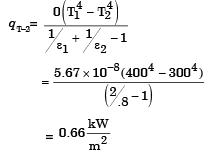QUESTION: 12

The total emissive power of a surface is 500 W/ m2 at temperature T1 and 1200 W/m2 at emperature T2, where the temperature are in Kelvin. Assuming the emissivity of the surface to be constant, the ratio of the temperature T1/T2 is



Solution:

Emissive power ∝ T4
⇒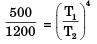∴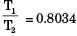QUESTION: 13

The emissive power of a blackbody is P. If its absolute temperature is doubled, the emissive power becomes



Solution:

Eb = σ T4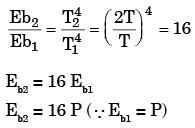QUESTION: 14

The peak wavelength of radiation emitted by a black body at a temperature of 2000 K is 1.45 μm. If the peak wavelength of emitted radiation changes to 2.90 μm , then the temperature (in K) of the black body is



Solution:

From wein’s displacement law
λmax T = constant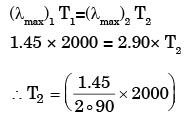T2 = 1000 K

QUESTION: 15

For the circular tube of equal length and diameter shown in figure below, the view factor F13 is 0.17. The view factor F12 in this case will be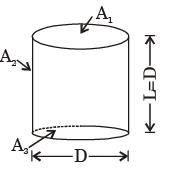Solution: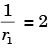and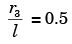F13 = 0.17,
F11 = 0,
F11 + F12 + F13 = 1
∴ F12 = 1 – F13
= 1 – 0 – 17 = 0.83

QUESTION: 16

A solid cylinder (surface 2) is located at the centre of a hollow sphere (surface 1). The diameter of the sphere is 1 m, while the cylinder has a diameter and length of 0.5 m each.'The radiation configuration factor F11 is



Solution: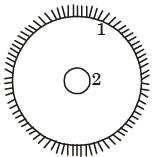Diameter of sphere = 1 m
Diameter of aylinder = 0.5 m
F 21 + F22 = 1 (∵ F22 = 0)
F21 = 1
we know that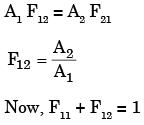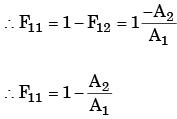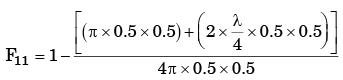= 0.625

QUESTION: 17

A hollow enclosure is formed between two inf initely long concentric cylinders of radii 1 m and 2 m, respectively. Radiative heat exchange takes place between the inner surface of the larger cylinder (surface-2) and the outer surface of the smaller cylinder (surface-1). The radiating surface are diffuse and the medium in the enclosure is non- participating. The fraction of the thermal radiation leaving the larger surface and striking itself is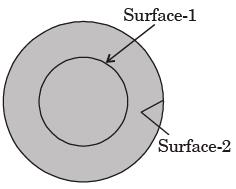Solution:

F 11 + F 12 = 1
F 21 + F 22 = 1
F 11 = 0 due to concave surface
F 12 = 1
Now A 1 F 12 = A 2 F 21
⇒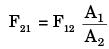⇒ F12 = 1
∴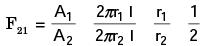F 21 = 0.5
F 22 = 1 – F 21 = 0.5

QUESTION: 18

Consider two infinitely long thin concentric tubes of circular cross section as shown in figure. If D1 and D2 are the diameters of the inner and outer tubes respectively, then the view factor F22 is given by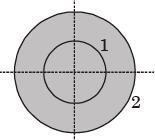Solution:

F22 = 1 – F21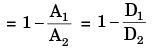QUESTION: 19

A solid sphere of radius r1 = 20 mm is placed concentrically inside a hollow sphere of radius r2 = 30 mm as shown in the figure.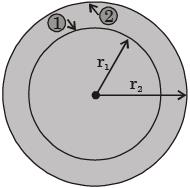The view factor F21 for radiation heat transfer is



Solution:

F 11 + F 12 =1, here F 11 = 0
∴ F 12 = 1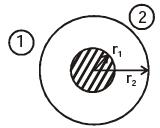Now from reciprocating law
A 1 F 12 = A 2 F 21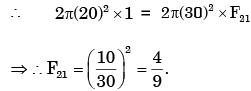QUESTION: 20

A solid sphere 1 of radius r is placed inside a hollow, closed hemispherical surface 2 of radius 4r. The shape factor F is,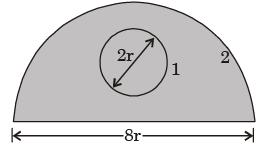Solution:

f11 + f12 = 1
∴ f12 = 1
f21 A2 = f12 A1
∴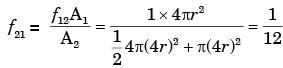QUESTION: 21

Consider the radiation heat exchange inside an annulus between two very long concentric cylinders. The radius of the outer cylinder is R0 and that of the inner cylinder is Ri. The radiation view factor of the outer cylinder onto itself is



Solution: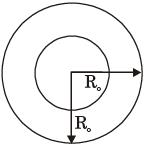View factor
F1– 1 = 0
F1– 2 = 1
Reciprocacy theorem
A 2F 2– 1 = A 1F 1– 2
⇒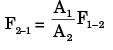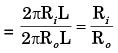F2– 1 + F2– 2 = 1
∴ F2– 2 = 1 – F2– 1 = 1 - Ri/Ro

QUESTION: 22

Sphere 1 with a diameter of 0.1 m is completely enclosed by another sphere 2 of diameter 0.4 m.The view factor F12 is



Solution: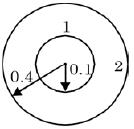F11 = 0
F12 = 1

QUESTION: 23

Two infinite parallel plates are placed at a certain distance apart. An infinite radiation shield is inserted between the plates without touching any of them to reduce heat exchange between the plates. Assume that the emissivities of plates and radiation shield are equal. The ratio of the net heat exchange between the plates with and without the shield is



Solution: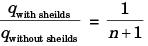here, n = 1
∴